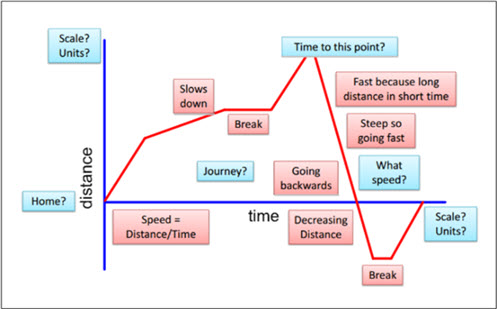Go to website

# Distance time graph

This sequence of lessons explores how well students understand the relationship between distance and time, through interpreting distance and time graphs. This is achieved by figuring out the displacement and acceleration of an object, determining speed and velocity. The lessons are accompanied by a PowerPoint and a student prompt sheet.

Year level(s) Year 7
Audience Teacher
Teaching strategies and pedagogical approaches Collaborative learning, Differentiated teaching, Feedback, Mathematics investigation, Questioning, Worked examples
Keywords distance, time, speed, veloctiy, displacement, acceleration, negative and positive acceleration

## Curriculum alignment

Curriculum connections Critical and creative thinking, English, Numeracy
Strand and focus Number, Build understanding, Apply understanding
Topics Addition and subtraction, Data representation and interpretation, Integers, Time, Using units of measurement
AC: Mathematics (V9.0) content descriptions
AC9M7A04
Describe relationships between variables represented in graphs of functions from authentic data

Numeracy progression Measuring time (P7)
Number patterns and algebraic thinking (P7)
Interpreting and representing data (P6)